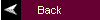MATLAB Tutorial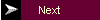4. Discrete-Time System Analysis

A. Convolution

Commands covered:

conv
deconv

To perform discrete time convolution, x[n]*h[n], define the vectors x and h with elements in the sequences x[n] and h[n]. Then use the command

y = conv(x,h)

This command assumes that the first element in x and the first element in h correspond to n=0, so that the first element in the resulting output vector corresponds to n=0. If this is not the case, then the output vector will be computed correctly, but the index will have to be adjusted. For example,

x = [1 1 1 1 1];
h = [0 1 2 3];
y = conv(x,h);

yields y = [0 1 3 6 6 6 5 3]. If x is indexed as described above, then y = 0, y = 1, .... In general, total up the index of the first element in h and the index of the first element in x, this is the index of the first element in y. For example, if the first element in h corresponds to n = -2 and the first element in x corresponds to n = -3, then the first element in y corresponds to n = -5.

Care must be taken when computing the convolution of infinite duration signals. If the vector x has length q and the vector h has length r, then you must truncate the vector y to have length min(q,r). See the comments in Problem 3.7 of the textbook for additional information.

The command conv can also be used to multiply polynomials: suppose that the coefficients of a(s) are given in the vector a and the coefficients of b(s) are given in the vector b, then the coefficients of the polynomial a(s)b(s) can be found as the elements of the vector defined by ab = conv(a,b).

The command deconv is the inverse procedure to the convolution. In this text, it is used as a means of dividing polynomials. Given a(s) and b(s) with coefficients stored in a and b, then the coefficients of c(s) = b(s)/a(s) are found by using the command c = deconv(b,a).

back to list of contents

B. Transfer Function Representation

For a discrete-time transfer function, the coefficients are stored in descending powers of z or ascending powers of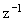. For example,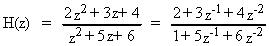then define the vectors as

num = [2 3 4];
den = [1 5 6];
back to list of contents

C. Time Simulations

Commands Covered:

recur
conv
dstep
dimpulse
filter

There are three methods to compute the response of a system described by the following recursive relationshipThe first method uses the command recur and is useful when there are nonzero initial conditions. This command is available from the MathWorks ftp site and a shortened version is given in Figure C.5 of the textbook. The inputs to the function are the coefficients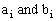stored in the vectors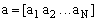and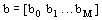, the initial conditions on x and on y are stored in the vectors x0 = [x[n0-M], x[n0-M+1],...,x[n0-1]] and y0 = [y[n0-N], y[n0-N+1],...,y[n0-1]]], and the time indices for which the solution needs to be calculated are stored in the vector n where n0 represents the first element in this vector. To use recur, type

y = recur(a,b,n,x,x0,y0);

The output is a vector y with elements y[n]; the first element of y corresponds to the time index n0. For example, consider the system described by

y[n] - 0.6y[n-1] + 0.08y[n-2] = x[n-1]

where x[n] = u[n] and with initial conditions y[-1] = 2, y[-2] = 1, and x[-1] = x[-2] = 0. To compute the response y[n] for n = 0, 1,...,10, type

a = [-0.6 0.08]; b = [0 1];
x0 = 0; y0 = [1 2];
n = 0:10;
x = ones(1,11);
y = recur(a,b,n,x,x0,y0);

The vector y contains the values of y[n] for n = 0,1,...,10.

The second method to compute the response uses convolution and is useful when the initial conditions on y are zero. This method involves first finding the impulse response of the system, h[n], and then convolving h[n] with x[n] as discussed in Section 4.A. For example, consider the system described above with zero initial conditions, that is, y[-1]=y[-2]=0. The impulse response for this system is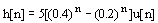. The commands to compute y[n] are

n = 0:10;
x = ones(1,11);
h = 5*(0.4).^n - 5*(.02).^n;
y = conv(x,h);
y = y(1:length(n));

The vector y contains the values of y[n] for n = 0,1,...,10. Note that the vector was truncated to length(n) because both x[n] and h[n] are infinite duration signals. See the comments in Section 4.A regarding the convolution of infinite duration signals.

The third method of solving for the response requires that the transfer function of the system be known. The commands dstep and dimpulse compute the unit step response and the unit impulse response, respectively while the command filter computes the response to initial conditions and to arbitrary inputs. The denominator coefficients are stored as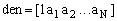and the numerator coefficients are stored as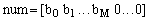where there are N-M zeros padded on the end of the coefficients. For example, consider the system given above with initial conditions y[-1] = y[-2] = 0. To compute the step response for n=0 to n=10, type the commands

n = 0:10;
num = [0 1 0]; den = [1 -0.6 0.08];
y = dstep(num,den,length(n));

The response can then be plotted using the stem plot. To compute the impulse response, simply replace dstep with dimpulse in the above commands.

To compute the response to an arbitrary input, store the input sequence in the vector x. The command

y = filter(num,den,x);

is used to compute the system response. If the system has nonzero initial conditions, the initial conditions can be stored in a vector v0. For a first order system where N=M=1, define. For a second order system where N=M=2, define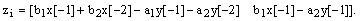. To compute the response with nonzero initial conditions, type

y = filter(num,den,x,zi);

For example, consider the previous system with the initial conditions y[-1] = 2 and y[-2] = 1 and input x[n] = u[n]. Type the following commands to compute y[n].

n = 0:10; x = ones(1:11);
num = [0 1 0]; den = [1 -0.6 0.08];
zi = [0.6*2-0.08*1, -0.08*2];
y = filter(num,den,x,zi);
back to list of contents

D. Frequency Response Plots

Commands covered:

freqz

The DTFT of a system can be calculated from the transfer function using freqz. Define the numerator and the denominator of the transfer function in num and den. The command

[H,Omega] = freqz(num,den,n,'whole');

computes the DTFT for n points equally spaced around the unit circle at the frequencies contained in the vector Omega. The magnitude of H is found from abs(H) and the phase of H is found from angle(H). To customize the range for W, define a vector Omega of desired frequencies, for example Omega = -pi:2*pi/300:pi defines a vector of length 301 with values that range from -p to p. To get the DTFT at these frequencies, type

H = freqz(num,den,Omega);

back to list of contents

E. Digital Filter Design

Commands covered:

bilinear
butter
cheby1
hamming
hanning

The analog prototype method of designing IIR filters can be done by first designing an analog filter with the desired characteristics as shown in Section 3.D, then mapping the filter to the discrete-time domain. Store the numerator and denominator of the analog filter, H(s), in the vectors num and den, and let T be the sampling period. Then the numerator and denominator of the digital filter H(z) is found from the following command

[numd,dend] = bilinear(num,den,1/T)

Alternately, the commands butter and cheby1 automatically design the analog filter and then use the bilinear transformation to map the filter to the discrete-time domain. Lowpass, highpass, bandstop, and bandpass filters can be designed using this method. The digital cutoff frequencies must be specified; these should be normalized by pi. To design a digital lowpass filter based on the analog Butterworth filter, use the commands:

[num,den] = butter(n,Omegac)

where n is the number of poles and Omegac is the normalized digital cutoff frequency,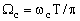. To design a highpass filter with cutoff frequency Omegac, use the commands

[num,den] = butter(n,Omegac,'high')

To design a bandpass filter with passband from Omega1 to Omega2, define Omega = [Omega1,Omega2] and use the command

[num,den] = butter(n,Omega)

To design a bandstop filter with stopband from Omega1 to Omega2, define Omega = [Omega1, Omega2] and use the command

[num,den] = butter(n,Omega,'stop')

The design for an nth order Type I Chebyshev filter is accomplished using the same methods as for butter except that "butter" is replaced by "cheby1":

[num,den] = cheby1(n,Omegac); % for a lowpass filter
[num,den] = cheby1(n,Omegac,'high'); % for a highpass filter

If Omega has two elements,

[num,den] = cheby1(n,Omega); % for a bandpass
[num,den] = cheby1(n,Omega,'stop'); % for a bandstop

The windows used in FIR filter design are given by

w = boxcar(N) % rectangular window
w = hamming(N)
w = hanning(N)

These commands are used to truncate the infinite impulse response of an ideal digital filter with the result being an FIR filter with length N.

The Signal Processing Toolbox also provides commands for computing the FIR filter directly. To obtain an FIR filter with length N and cutoff frequency Omegac (normalized by pi) use the command

h = fir1(N-1,Omegac)

The vector h contains the impulse response of the FIR where h(1) is the value of h.

A length N highpass filter with normalized cutoff frequency Omegac is designed by using the command

h = fir1(N-1,Omegac,'high')

A bandpass with passband from Omega1 to Omega2 is obtained by typing

h = fir1(N-1,Omega)

where Omega = [Omega1,Omega2]. A bandstop filter with stopband from Omega1 to Omega2 is obtained by typing

h = fir1(N-1,Omega,'stop')

where Omega = [Omega1,Omega2]. The fir1 command uses the Hamming window by default. Other windows are obtained by adding an option of 'hanning' or 'boxcar' to the arguments; for example,

h = fir1(N-1,Omegac,'high',boxcar(N))

creates a highpass FIR filter with cutoff frequency Omegac using a rectangular window.

back to list of contents

F. Digital Control Design

Commands covered:

bilinear
c2dm
hybrid

An analog controller G(s) can be mapped to a digital controller D(z) using the bilinear transformation or the step response matching method. Store the numerator and denominator of G(s) in num and den. Then the numerator and denominator of D(z) is found from the bilinear transformation using the commands

[numd,dend] = bilinear(num,den,1/T)

where T is the sampling frequency. To use the step invariant method, use the commands

[numd,dend] = c2dm(num,den,T,'zoh')

To simulate the response of a continuous-time plant G(s) with a digital controller D(z), use the command hybrid. Consider the block diagram in Figure 11.25 of the text. The numerator and denominator coefficients of the plant are stored in numG and denG; the numerator and denominator coefficients of the controller are stored in numD and denD; the reference input signal is stored in r; and the sampling time is stored in T. The increments in the time vector should selected to be the sampling time divided by an integer, for example, t = 0:b:Tend where there is some integer m such that bm=T. The command is used as

[y,ud] = hybrid(numG,denG,numD,denD,T,t,r);

The outputs of the command are the system response, y, and the control signal that is input to the plant, ud. The M-file contains a loop which computes the discrete-time control and then simulates the continuous-time plant for T seconds with the constant control. The process repeats for the next T second interval.

G. State Space Representation

Commands Covered:

dlsim
dstep
dimpulse

Most of the commands for the continuous time state space representation also work for the discrete time state space. For example, ss2tf, tf2ss, and ss2ss for discrete time are used exactly the same way as for the continuous time case discussed in Section 3.F. There is a discrete time version of the command lsim, which is used as follows:

[y,x] = dlsim(A,B,C,D,u,n);

where the output is stored in y, the states are stored in x, the input is stored in u and the time index is stored in n.

This tutorial is available as a supplement to the textbook Fundamentals of Signals and Systems Using Matlab by Edward Kamen and Bonnie Heck, published by Prentice Hall. The tutorial is designed for students using either the professional version of MATLAB (ver. 5.0) with the Control Systems Toolbox (ver. 4.0) and the Signal Processing Toolbox (ver. 3.0), or using the Student Edition of MATLAB (ver. 4.0). For more information on MATLAB, contact The Mathworks, Inc.